Next: 3.2 The statistical basis of entropy Previous: 3. The statistical theory of thermodynamics

# 3.1 Microstates and Macrostates

Take-home message: The properties of a macrostate are averaged over many microstates.

Classical thermodynamics describes macroscopic systems in terms of a few variables (functions of state): temperature, pressure, volume... But such a system is really made of atoms, so a much richer description must be possible in principle: we could specify the quantum state of all the atoms--the microstate. Of course as the atoms interact this state changes very rapidly-perhaps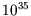times a second. But the observed macrostate doesn't change. Many different microstates all correspond to the same macrostate.

This suggests we can calculate the macroscopic behaviour of the system by averaging over the corresponding microstates. We can derive thermodynamics from the quantum behaviour of atoms and molecules.

Of course only a few systems are really fully calculable: those in which the interactions between atoms are particularly simple--or absent. We will be able to tackle the ideal paramagnet and the ideal gas, including the latter at densities so high that the quantum nature of the system becomes important. The van der Waals gas and a paramagnet with nearest neighbour interactions can be worked out, as can crystalline solids with simple defects. Going beyond that is tough. But the proof-of-principle that these give is compelling.

The crucial link from microscopic to macroscopic properties is as follows. If the value of some quantity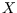in the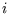th microstate is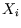, and the probability that the system is in that microstate is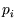, then the value ofin the macrostate is the ensemble average (more details here.)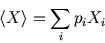We start by considering an isolated system (constant energy, volume and particle number). The fundamental principle that allows the averaging over microstate to be done is the postulate of equal a priori probabilities or, in plain English, the assumption that all allowed microstates are equally likely. (Allowed or accessible means having the same volume, particle number and and total energy as the macrostate.) We use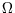for the number of such microstates, so the probability of the system being in any one microstate is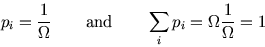The idea of microstates is explored in a simple example here.

References

• Mandl 2.1-2
• Bowley and Sánchez 4.1 (beware-B&S use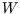for)
• Kittel and Kroemer 1,2

SubsectionsNext: 3.2 The statistical basis of entropy Previous: 3. The statistical theory of thermodynamics
Judith McGovern 2004-03-17